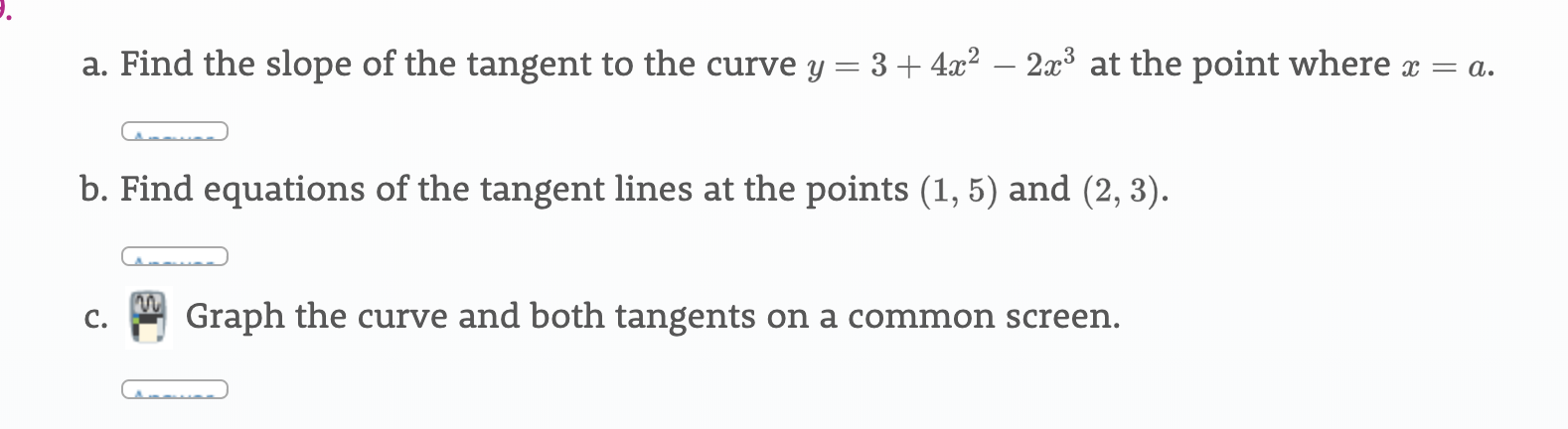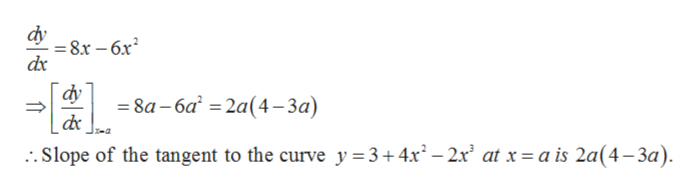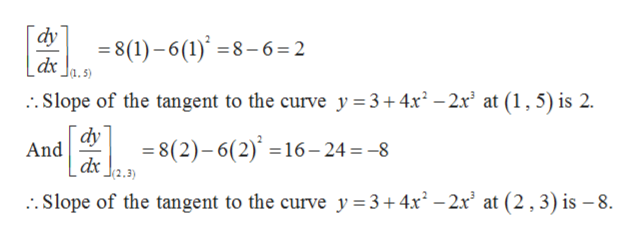a. Find the slope of the tangent to the curve y = 3 + 4x2 - 2x3 at the point where x = ab. Find equations of the tangent lines at the points (1, 5) and (2, 3)Graph the curve and both tangents on a common screen.C.

Questionhelp_outlineImage Transcriptionclosea. Find the slope of the tangent to the curve y = 3 + 4x2 - 2x3 at the point where x = a b. Find equations of the tangent lines at the points (1, 5) and (2, 3) Graph the curve and both tangents on a common screen. C. fullscreen
Step 1

Given curve is,

Step 2

a). The slope of the tangent is the derivative of the given curve at the given point x = a.

Therefore,help_outlineImage Transcriptionclosedy =8x - 6x2 dx dy =8a-6a 2a (4-3a) .Slope of the tangent to the curve y 3+4x2- 2x2 at x = a is 2a(4-3a) 1 fullscreen
Step 3

b). given points are ( 1, 5) and (2, 3).

First we find the slope of the ta...help_outlineImage Transcriptionclosedy 8(1)-6(1)8-6=2 dr ..Slope of the tangent to the curve y = 3+4x2 -2x' at (1,5) is 2. dy And 8(2)-6(2)16-24 -8 . Slope of the tangent to the curve y 3+ 4x2 -2x' at (2,3) is - 8. fullscreen

Want to see the full answer?

See Solution

Want to see this answer and more?

Our solutions are written by experts, many with advanced degrees, and available 24/7

See Solution
Tagged in

Derivative